# Study of a function

By a.zampa
• Period: 100 to 199

## Determining the domain

The (natural) domain of a function is the set of all real numbers to which the function assigns a value
• 150

# Solve inequalities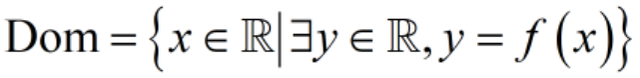You need to impose the (existence) conditions under which each operation (such as division, square root, ...) or function (such as logarithms, ...), present in the analytic expression of the given function, can have a value (e.g. you can't divide by 0, you can't take the square root of a negative number, ...)
• Period: 200 to 299

## Determining symmetries

Determine if the graph of the function is invariant under some type of geometric transformation
• 233

# Parity (axial / central symmetry)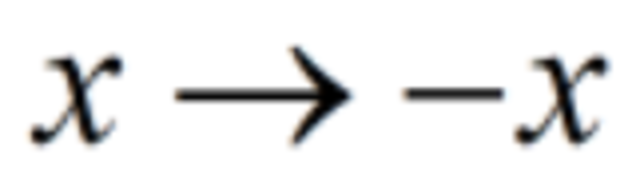The function is even if its graph is symmetric with respect to the ordinate axis, i.e. the value of the function is invariant under change of sign of the independent variable The function is odd if its graph is symmetric with respect to the origin of coordinate axis, i.e. the value of the function changes sign under change of sign of the independent variable
• 267

# Periodicity (translation)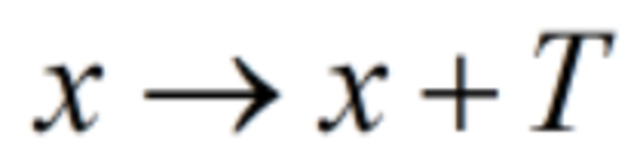The function is periodic, with period T, if its graph is invariant under horizontal translation by an amount equal to T, i.e. its value does not change when adding T to the independent variable
• Period: 300 to 399

## Determining sign and intersections of the graph with coordinate axes

Determine the regions of the cartesian plane wher the graph of the fuction is placed
• 325

# Intersection with the axis of ordinates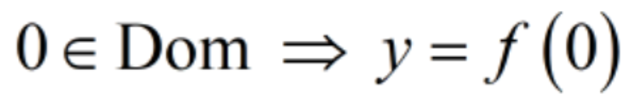If 0 is an element of the domain then the graph intersect the axis of ordinates at the point whose ordinate is the value associated to 0 by the function
• 350

# Intersections with the axis of abscissas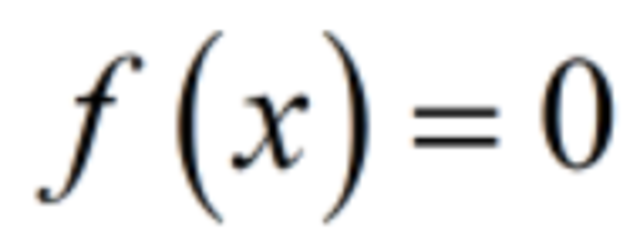The intersections of the graph with the axis of abscissas correspond to the zeroes of the function: to find them you need to solve an equation
• 375

# Sign of the function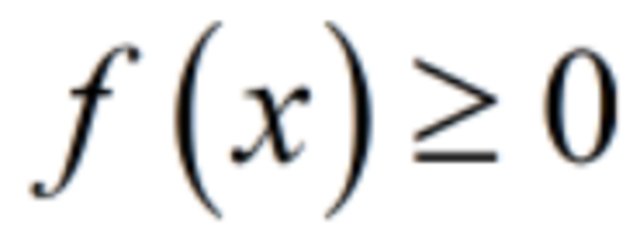The sign of the function allows to know if the corresponding point of the graph is above or below the axis of abscissas. You can obtain the set of positivity and the set of negativity of the function (together with the set of zeroes) by solving a single inequality
• Period: 400 to 499

## Classifying discontinuities/singularities

Analyse the behaviour of the function near the accumulation points of its domain where it is not continuous or undefined by means of limits
• 425

# Jump discontinuty / singularity (of the first kind)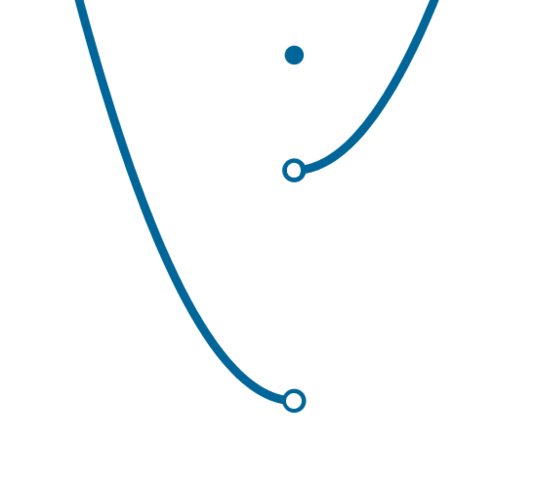The left and right limits are different real numbers
• 450

# Essential discontiunity / singularity (of the second kind)At least one among the left and right limits is infinite or does not exist
• 475

# Removable discontinuity / singularity (of the third kind)The left and the right limit are the same real number
• Period: 500 to 599

## Determining asymptotes

Analyse the behaviour of the graph at infinity to check if it approximates some stright line
• 525

# Vertical asymptoteThe graph of a function has a vertical asymptote if and only if the limit of the function when the independent variable tends to a real number is infinite
• 550

# Classifying inflection pointsHorizontal asymptoteThe graph of the function has anhorizontal asymptote if the limit of the function when the independent variable tends to infinity is a real number
• 575

# Slant asymptote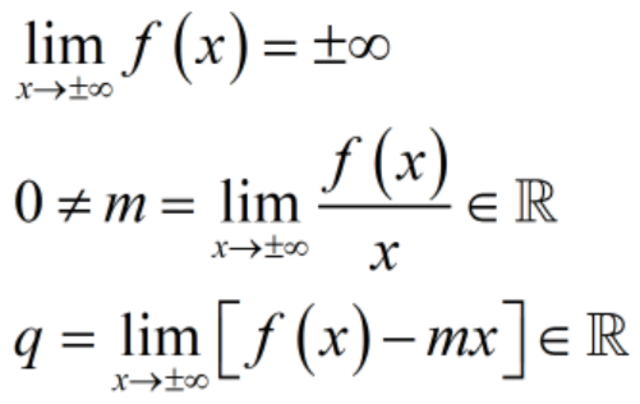For a slant asymptote to the graph of a function to exists three conditions need to be met
• Period: 600 to 699

## Determining growth (monotonicity)

Find the regions of the domain where the function is increasing or decreasing
• 625

# Calculate the (first) derivativeThe (first) derivative of the function hosts information about the slope of the line tangent to the graph, hence the study of the monotonicity of the function is performed by solving a suitable inequality
• 650

# Increasing functionThe function is increasing in every interval of its domain where the sign of its (first) derivative is positive
• 675

# Decreasing function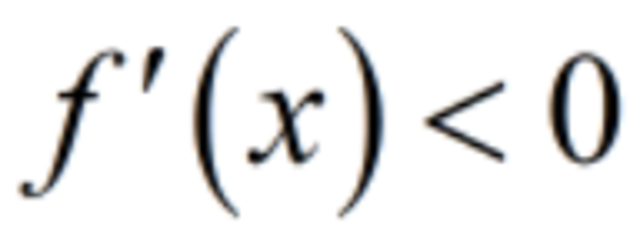The function is decreasing in every interval of its domain where the sign of its (first) derivative is negative
• Period: 700 to 799

## Classifying stationary (critical) points

Determine the position of estremals and of inflection points with horizontal tangent
• 720

# Critical pointsZeroes of the (first) derivative are called critical (or stationary) points: they correspond to points of the graph where the tangent line is horizontal and generally, but not always, they signal a change of monotonicity (in which case they are also called extrema)
• 740

# Local maximumA local maximum, which is one f the two inds of extremum, is a critical point for which there exist a left neighbourhood where the (first) derivative of the function is positive, and a right neighbourhood where the derivative is negative
• 760

# Local minimumA local minimum, which is one f the two kinds of extremum, is a critical point for which there exist a left neighbourhood where the (first) derivative of the function is negative, and a right neighbourhood where the derivative is positive
• 780

# Horizontal point of inflectionA horizontal point of inflection is a critical point which has a punctured neighbourhood where the (first) derivative has constant sign, i.e. it is either positive in that neighbourhood, or it is negative in that neighbourhood
• Period: 800 to 899

## Classifying points of non-derivability

Understand the "branches" of th graph near points where the function is continuous but it is not derivable
• 825

# Corner point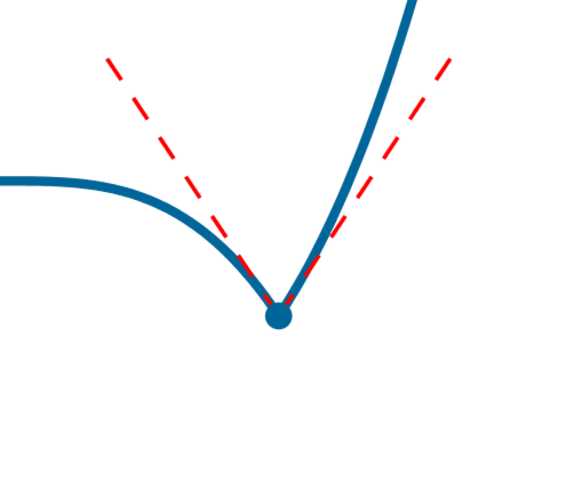A corner point is a (not isolated) point of continuity of the function where the left and right limits of the (first) derivative are distinct real numbers
• 850

# Cusp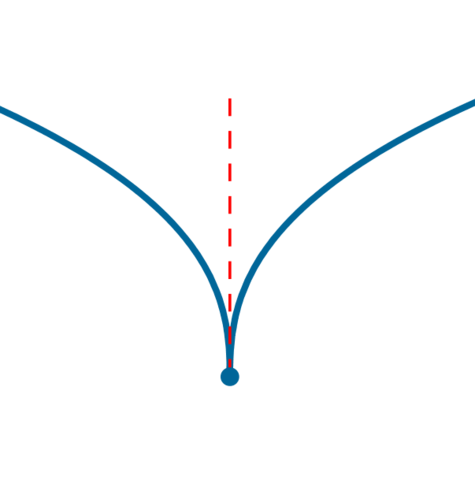A cusp is a (not isolated) point of continuity of the function where the left and right limits of the (first) derivative are infinite with different signs
• 875

# Vertical point of inflectionA vertical point of inflection is a (not isolated) point of continuity of the function where the left and right limits of the (first) derivative are infinite with the same sign
• Period: 900 to 999

## Determining the concavity

Find in which way the graph curves with respect to the tangent line
• 925

# Calculate the second derivativeThe second derivative of the function hosts information about the concavity of the graph, i.e. about which side of the tangent line the graph stays in a neighbourhood of the point. The function is concave up, or convex, at a point in a punctured neighbourhood of which the graph is above of the tangent line, whereas if the graph is below of the tangent line there the function is concave down, or concave. The study of the concavity of the function is performed by solving a suitable inequality
• 950

# Concave up (convex) functionThe function is concave up (convex) in every interval of its domain where the sign of its second derivative is positive
• 975

# Concave down (concave) function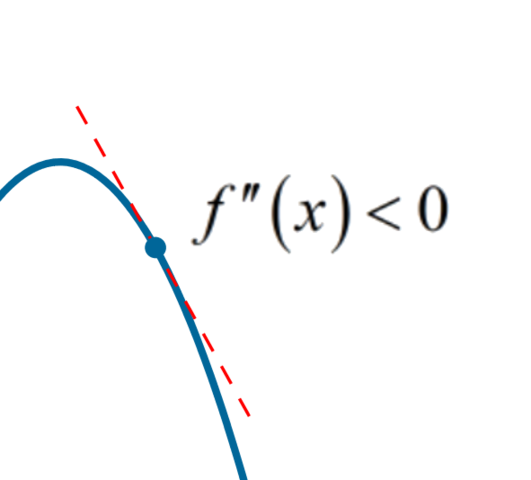The function is concave down (concave) in every interval of its domain where the sign of its second derivative is negative
• Period: 1000 to 1099

## Classifying inflection points

Determine the type of the points whhere the graph changes its concavity type
• 1025

# Points of inflection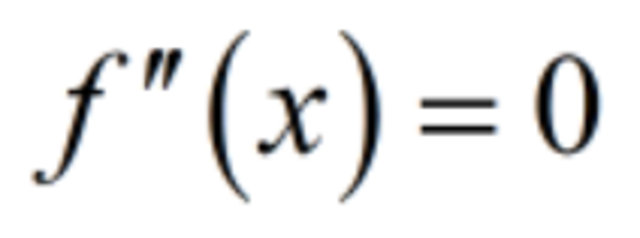Zeroes of the second derivative signal a possible change of behaviour of the function with respect to concavity. If the sign of the second derivative changes crossing a zeroe then the corresponding point of the graph is called apoint of inflection (the graph "flexes" at that point)
• 1050

# Ascending point of inflectionAn ascending point of inflection is a point for which there exist a left neighbourhood where the second derivative of the function is negative, and a right neighbourhood where the second derivative is positive. Therefore, locally the function is concave to the left of a descending point of inflection, while it is convex to its right
• 1075

# Descending point of inflectionA descending point of inflection is a point for which there exist a left neighbourhood where the second derivative of the function is positive, and a right neighbourhood where the second derivative is negative. Therefore, locally the function is convex to the left of a descending point of inflection, while it is concave to its right
• 1100

# Drawing the probable graphAll the information collected during the study of the function allows to sketch a probable graph representing geometrically the main properties of the function